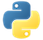# MeanPydoc

Transforms for computing the arithmetic mean of the elements in a collection, or the mean of the values associated with each key in a collection of key-value pairs.

## Examples

In the following example, we create a pipeline with a `PCollection`. Then, we get the element with the average value in different ways.

### Example 1: Mean of element in a PCollection

We use `Mean.Globally()` to get the average of the elements from the entire `PCollection`.

``````import apache_beam as beam

with beam.Pipeline() as pipeline:
mean_element = (
pipeline
| 'Create numbers' >> beam.Create([3, 4, 1, 2])
| 'Get mean value' >> beam.combiners.Mean.Globally()
| beam.Map(print))``````

Output:

``2.5``View source code

### Example 2: Mean of elements for each key

We use `Mean.PerKey()` to get the average of the elements for each unique key in a `PCollection` of key-values.

``````import apache_beam as beam

with beam.Pipeline() as pipeline:
elements_with_mean_value_per_key = (
pipeline
| 'Create produce' >> beam.Create([
('🥕', 3),
('🥕', 2),
('🍆', 1),
('🍅', 4),
('🍅', 5),
('🍅', 3),
])
| 'Get mean value per key' >> beam.combiners.Mean.PerKey()
| beam.Map(print))``````

Output:

``````('🥕', 2.5)
('🍆', 1.0)
('🍅', 4.0)``````View source codePydoc